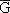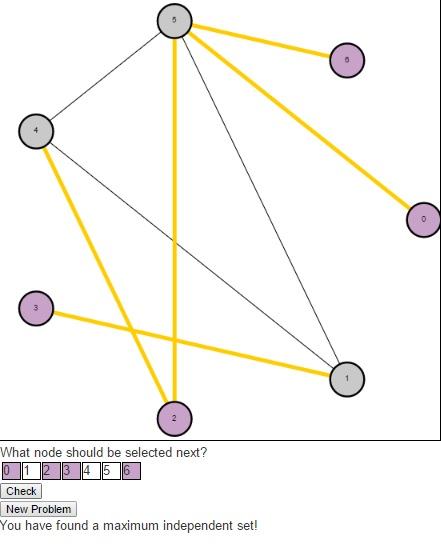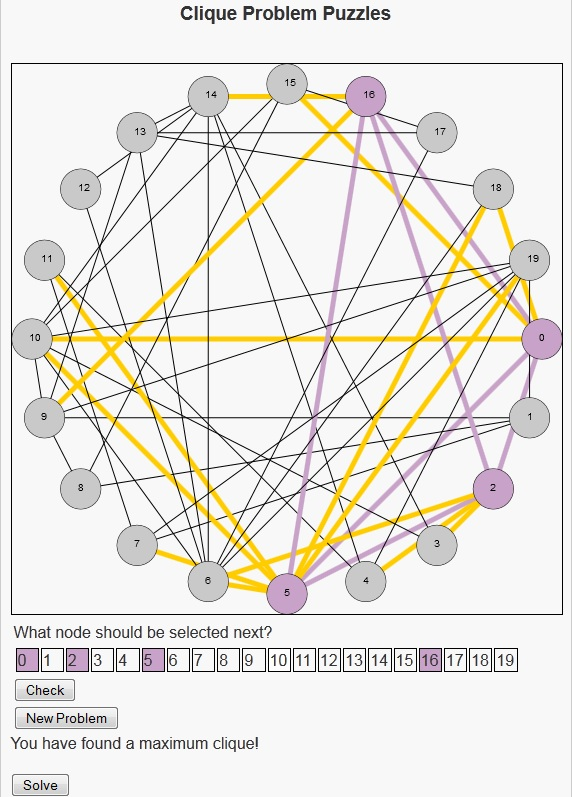# Independent Set Puzzles

In this post, I want to return to the idea of NP-Complete problems. There is a more technical, more formal definition that I can refer you to, but I like to refer to the images from Garey and Johnson’s “Computers and Intractability: A Guide to the Theory of NP Completeness”. The images helps to understand the difficulty of NP-Complete problems by presenting two images. The first image shows a single individual speaking to someone saying that he has been unable to solve the problem. The second image shows that same individual speaking to the same person behind the desk, but saying that not only was he unable to solve the problem but neither was a long line of people. The theory of NP Complete problems revolves around the concept that if an efficient algorithm exists for an NP-Complete problem, then an efficient algorithm exists for all problems in the class NP.

Today, I would like to present a puzzle I created to play with the Independent Set problem. This is the problem where we are given a graph G = (V, E) and are asked to find a maximum set of vertices S such that there is no edge in the graph G between any two vertices in S. The decision version (the problem asking whether there is an independent set of size k) of this problem is NP-Complete, so the known algorithms for problem either have a slow running time, or do not solve it exactly.

This problem is very related to another puzzle I posted last year called the clique problem. In fact, Karp originally proved that Clique was NP-Complete by showing that if Clique could be solved efficiently, then Independent Set could be solved efficiently. He did this by constructing a second graph [G bar], called the compliment of G (containing the same vertices in G, along with the edges that are not present in G. Any edge present in G will not be present in). Then he showed that the nodes representing a maximum clique in G would represent a maximum independent set in. He had already shown that Independent Set was NP-Complete, which meant that both Independent Set and Clique were among the most difficult problems within the class known as NP.The puzzle begins with an undirected graph and asks users to find a maximum independent set. Users should click on the numbers in the table below the graph indicating the nodes they wish to select in their independent set (purple indicates that the node is selected, gray indicates that it is not). Once a user have a potential solution, they can press the “Check” button to see if their solution is optimal. If a user is having trouble and simply wishes to see the maximum independent set, they can press the “Solve” button. And to generate a new problem, users can press the “New Problem” button.As a result of this relationship between the Clique problem and the Independent Set problem, the Bron-Kerbosch algorithm that was used to find maximum cliques previously can also be used here.

# Clique Problem Puzzles

I still remember how I felt when I was first introduced to NP-Complete problems. Unlike the material I had learned up to that point, there seemed to be such mystery and intrigue and opportunity surrounding these problems. To use the example from Garey and Johnson’s book “Computers and Intractability: A Guide to the Theory of NP Completeness”, these were problems that not just one researcher found difficult, but that a number of researchers had been unable to find efficient algorithms to solve them. So what they did was show that the problems all had a special relationship with one another, and thus through this relationship if someone were to discover an algorithm to efficiently solve any one of these problems they would be able to efficiently solve all the problems in this class. This immediately got my mind working into a world where I, as a college student, would discover such an algorithm and be mentioned with the heavyweights of computer science like Lovelace, Babbage, Church, Turing, Cook, Karp and Dean.

Unfortunately I was a student so I did not have as much time to devote to this task as I would have liked. In my spare time though I would try to look at problems and see what kind of structure I found. One of my favorite problems was, The Clique Problem. This is a problem where we are given an undirected graph and seek to find a maximum subset of nodes in this graph that all have edges between them, i.e. a clique (Actually the NP-Complete version of this problem takes as input an undirected graph G and an integer k and asks if there is a clique in G of size k).

Although I now am more of the mindset that there do not exist efficient algorithms to solve NP-Complete problems, I thought it would be a nice project to see if I could re-create this feeling – both in myself and others. So I decided to write a program that generates a random undirected graph and asks users to try to find a maximum clique. To test users answers, I coded up an algorithm that works pretty well on smaller graphs, the Bron-Kerbosch Algorithm. This algorithm uses backtracking to find all maximal cliques, which then allows us to sort them by size and determine the largest.Users should click on the numbers in the table below the canvas indicating the nodes they wish to select in their clique (purple indicates that the node is selected, gray indicates that it is not). Once they have a potential solution, they can press the “Check” button to see if their solution is optimal. If a user is having trouble and simply wishes to see the maximum clique, they can press the “Solve” button. And to generate a new problem, users can press the “New Problem” button.

So I hope users have fun with the clique problem puzzles, and who knows maybe someone will discover an algorithm that efficiently solves this problem and become world famous.

# What is a “Hard” Problem?

Throughout our lives, we are introduced to a wide variety of problems. Naturally we tend to think of some problems as more difficult than others. If you were doing the exercises at the end of a chapter in a book, the author generally tends to begin with those exercises that can be completed in a few minutes or directly from the material in the chapter. The exercises in the end tend to be more time consuming and possibly require outside resources. In this sense, these authors tend to compare the difficulty of problems by the amount of time they expect the average reader to take to solve them.

This is a popular way to look at difficulty – in terms of how difficult it is for not only a single person to solve a problem (say you or I), but how long it would take our peers as well as yourself to solve the problem. But how can we measure this? Should I assume that because a problem takes me two years to solve that it would take the average person the same amount of time? Or, if a problem has never been solved, is that because of the difficulty of the problem or could it be for another reason like because no-one had thought of the problem before?

In particular, these questions were being asked in the world of computer science in the 1960s and 1970s. It was around this time that Stephen Cook came up with the concept being able to compare how difficult one problem was in comparison to another. A problem A can be “reduced” to another problem B if a way to solve problem B quickly also gives way to a way to solve problem A quickly.

This concept of reducibility provided a foundation for this concept of difficulty as it was no longer enough to say “I think this problem is hard”, or “the average person would take just as long as me to solve this problem”. No, instead, Cook considered problems that many thought were difficult and showed that the satisfiability problem (the question of whether a given boolean formula contains an assignment to the variables that satisfies all the clauses) could be used to show that all quickly verifiable decision problems (also called problems in NP), where the instances with a “yes” answer have short proofs of the fact that the answer is indeed “yes” could be reduced to this problem.

This meant that the satisfiability problem was at least as hard as every one of these quickly verifiable decision problems, so if this problem could be solved quickly, then every one of these quickly verifiable decision problems could also be solved quickly. So the satisfiability problem is at least as hard as every problem in this class, NP. We call such problems that have this property NP-Hard. Decision Problems that are NP-Hard are called NP-Complete. Richard Karp later published a paper that proposed 21 NP-Complete problems, one of which was the Knapsack Problem I spoke about last time.This concept of NP-Complete gives light to the image that became famous in the book by Garey and Johnson “Computers and Intractability: A Guide to the Theory of NP-Completeness”. It shows that the concept of difficulty still builds on the ability of our peers to solve a given problem. But by introducing a class of “hard” problems, and a litmus test for inclusion into that class, researchers could now consider new problems and determine their difficulty by comparing it to known hard problems.

(a quick note. I’ve used the term “quickly” here, but the formal phrase that I mean by that is that the problem is solvable by an algorithm whose worse case running time is bounded by a polynomial on the input parameters of the problem)

Other Blogs that have covered this topic:
Explorations
To Imaging, and Beyond!